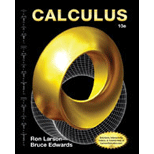# The functions f , g that satisfy the conditions lim x → 5 f ( x ) = 0 , lim x → 5 g ( x ) = 0 and lim x → 5 f ( x ) g ( x ) = 10 .### Calculus

10th Edition
Ron Larson + 1 other
Publisher: Cengage Learning
ISBN: 9781285057095### Calculus

10th Edition
Ron Larson + 1 other
Publisher: Cengage Learning
ISBN: 9781285057095

#### Solutions

Chapter 8.7, Problem 63E

(a)

To determine

## The functions f,g that satisfy the conditions limx→5f(x)=0, limx→5g(x)=0 and limx→5f(x)g(x)=10.

(b)

To determine

(c)

To determine

### Want to see the full answer?

Check out a sample textbook solution.

### Want to see this answer and more?

Experts are waiting 24/7 to provide step-by-step solutions in as fast as 30 minutes!*

*Response times may vary by subject and question complexity. Median response time is 34 minutes for paid subscribers and may be longer for promotional offers.